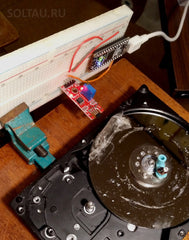# ⚙️ How to connect the hall sensor 49E to Arduino

The article discusses the connection of the Hall sensor 49E to the Arduino.

We will need:
• Arduino UNO or another compatible motherboard (I will use Arduino Nano);
• 49E module with Hall sensor;
• connecting wires (I recommend this set);

Description Hall sensor 49E

The Hall sensor is a device that records the change in magnetic field strength. At present, Hall-based sensors are widely used. For example:

rotational speed sensors are widely used in the automotive industry and everywhere where it is required to determine the speed of rotation of a wheel or other rotating object; Hall-based sensors came to replace mechanical reed switches;
proximity sensors; A typical example is a folding case on your smartphone, which turns on the screen backlight when opening;
rotation angle measurement;
measurement of vibration;
Magnetic field measurement - magnetometers and digital compasses;
current measurement (AC and DC);
measurement of air gaps, fluid levels, and many others.
You can purchase such a sensor here.

Module wiring diagram

The module with a Hall sensor contains the following components: a trimming resistor, a two-channel comparator, several matching resistors, a pair of LEDs, and the Hall sensor 49E itself.The trimmer resistor is used to adjust the sensitivity of the Hall sensor. The first LED indicates the presence of supply voltage on the module, the second indicates that the magnetic field has exceeded the set threshold.

The module with the sensor has 4 outputs. The purpose of the conclusions given in the table. In the third column of the table - the corresponding output of the Arduino board to which the module will be connected.

Output Purpose Arduino Output
G Ground. GND
+ Power supply +2,3…10 V. 5V
AO Analog output - the value of the magnetic field. A0
DO Digital output - an indicator of the excess of the magnetic field of a given threshold. 12

This is how the module with a Hall sensor connected to the Arduino Nano board will look like:Sketch for reading Hall sensor 49E

So let's check our sensor in action. We write the simplest sketch, which only does what displays the read values to the serial port.

```int analogPin = A0;
int digitalPin = 12;

void setup() {
pinMode(digitalPin, INPUT);
Serial.begin(9600);
}

void loop() {
Serial.println((String)digitalValue + "\t" + (String)analogValue);
delay(100);
}```

Load the sketch and see what is displayed in the port:Very interesting. The digital channel output is clear: "0" is the magnetic field below the value specified by the trimming resistor, "1" is higher (I brought the magnet to the sensor). And what does the analog channel show? Let's deal with this a little later.

Sketch to determine the speed of rotation of the disk

In order to determine the speed of rotation, we will use the signal from the digital channel of the sensor. Such a scheme is useful, for example, to create a speedometer for a bicycle.

For the demonstration, we will assemble such an installation: place the motionless Hall sensor (hold down the vice), and fix a permanent magnet on the surface of the rotating disk. As a rotating platform, I will have an old hard disk on which a magnet will be fixed with scotch tape (sorry for the lack of aesthetics).Recall the angular velocity formula:

ω = φ / t

where ω is the angular velocity, φ is the angle of rotation, t is the time for which the disk turned to this angle. In our case, the angle (1 turn) will be equal to 360 ° or 2π radians. All that remains for us is to calculate the time over which one revolution of the disk takes place.

In the sketch, we will catch the signal transition from the sensor from HIGH to LOW and calculate the difference between two consecutive transitions.

To determine the time interval, we use the millis() built-in function, which returns the number of milliseconds that have passed since the Arduino board was turned on.

```int digitalPin = 12;
unsigned long runTime;
int prevValue = 0;

void setup() {
pinMode(digitalPin, INPUT);
Serial.begin(9600);
runTime = millis();
}

void loop() {
delay(50);
if ((prevValue == HIGH) && (digitalValue == LOW)) {
unsigned long timeSpan = millis() - runTime;
runTime = millis();
Serial.println("Turnover period = " + (String)timeSpan + " ms");
double omega = 2 * PI / (timeSpan * 1.0E-3);
Serial.println("Angular velocity = " + (String)omega + " rad/s");
}
prevValue = digitalValue;
}```

Load the sketch, and start spinning our disk with a magnet. The turnover period and the angular velocity are displayed in the console window:

By the way, if two magnets are placed one after another on a disk, then it will be possible to determine not only the rotation speed but also the direction. Naturally, the sketch will have to complicate a little.

Returning to the idea of ​​a speedometer for a bicycle, you need to recall another formula - the relationship of angular and linear velocities:

v = ω r

Here, v is the linear velocity, ω is the angular velocity, r is the radius of the bicycle wheel. Now it is easy to finish our last sketch, taking into account this formula.

Values ​​from the analog channel of the Hall sensor 49E

Now let's see what the analog values ​​from the Hall sensor show.

The sensor produces a voltage that varies depending on the magnitude of the magnetic field. The magnetic field induction vector is measured in Gauss (G, GS in English). According to the technical description of the Hall detector, the measuring range of the Hall sensor is 49E ± 1500 Gs with a linear range from −1200 to +1200 Gs., And the sensitivity of the sensor is about 2.9 mV / Gs.